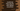# JavaScript program to find the element with highest occurrence in an array## Introduction :

In this tutorial, we will learn how to find the highest occurrence elements from an array in JavaScript. I will show you three different ways to solve this problem. Go through the examples and drop one comment below if you have any queries.

## Problem statement :

One array is given. We will take one integer array with all examples. You need to write one program to find out the maximum occurrence element or number in that array.

For example, if the array is :

``[1,2,3,4,1,1,2,3]``

The program will print 1, as three 1s are there in this array and that is the highest.

## Method 1: Using a loop :

``````// 1
const givenArray = [1, 2, 3, 4, 1, 1, 2, 3, 4];

// 2
let itemsMap = {};
let maxValue = 0;
let maxCount = 0;

// 3
for (let item of givenArray) {
// 4
if (itemsMap[item] == null) {
itemsMap[item] = 1;
} else {
itemsMap[item]++;
}

//5
if (itemsMap[item] > maxCount) {
maxValue = item;
maxCount = itemsMap[item];
}
}

// 6
console.log(`Value : \${maxValue}, Count : \${maxCount}`);``````

### Explanation :

The commented numbers in the above example denote the step numbers below :

1. givenArray is the array given. We are finding the element with the highest occurrence in this array.
2. itemsMap is an empty object. This object will be used to hold the element and its occurrence count as a key-value pair.
3. Iterate through the array elements one by one.
4. Check if the current element is on the map or not. If not, add this element as key and assign 1 as its value. This means this element occurs one time until now. Else, increment the current value.
5. If the value for the current element is more than maxCount, assign this element to maxValue and assign its count to maxCount.

It will print the below output :

``Value : 1, Count : 3``

## Method 2: Using reduce and filter:

``````const givenArray = [1, 2, 3, 4, 1, 1, 2, 3, 4];

const maxValue = givenArray.reduce((previous, current, i, arr) => {
if (
arr.filter(item => item === previous).length >
arr.filter(item => item === current).length
) {
return previous;
} else {
return current;
}
});

console.log(`Maximum occurrence value : \${maxValue}`);``````

reduce() method gives one result from an array by operating on its items using a function. The callback function provides the previous and the current element. In our case, we are checking the amount of time the previous item occurs in the array and the amount of time the current item occurs. We are using filter for that. Based on that, we are returning the item that is occurred maximum time. It will return to us the maximum time occurred element finally.

You can also write the above program like below :

``````const givenArray = [1, 2, 3, 4, 1, 1, 2, 3, 4];

const maxValue = givenArray.reduce((previous, current, i, arr) =>
arr.filter(item => item === previous).length >
arr.filter(item => item === current).length
? previous
: current
);

console.log(`Maximum occurrence value : \${maxValue}`);``````

## Method 3: Using sort :

sort method sorts the array elements based on one function. We can use this method to find the number with maximum occurrence like below :

``````const givenArray = [1, 2, 3, 4, 1, 1, 2, 3, 4];

const maxValue = givenArray
.sort(
(previous, current) =>
givenArray.filter(item => item === previous).length -
givenArray.filter(item => item === current).length
)
.pop();

console.log(`Maximum occurrence value : \${maxValue}`);``````

It will push the elements with the highest occurrence to the end. We are using pop() to get the last element i.e. the number with maximum occurrence in the array.